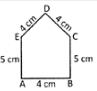Courses

# Test : Area, Perimeter And Volume - 1

## 10 Questions MCQ Test Mathematics for Class 5 (V) - CBSE and NCERT Curriculum | Test : Area, Perimeter And Volume - 1

Description
This mock test of Test : Area, Perimeter And Volume - 1 for Class 5 helps you for every Class 5 entrance exam. This contains 10 Multiple Choice Questions for Class 5 Test : Area, Perimeter And Volume - 1 (mcq) to study with solutions a complete question bank. The solved questions answers in this Test : Area, Perimeter And Volume - 1 quiz give you a good mix of easy questions and tough questions. Class 5 students definitely take this Test : Area, Perimeter And Volume - 1 exercise for a better result in the exam. You can find other Test : Area, Perimeter And Volume - 1 extra questions, long questions & short questions for Class 5 on EduRev as well by searching above.
QUESTION: 1

Solution:
QUESTION: 2

Solution:
QUESTION: 3

### Base of a triangle is 4 cm less than its height. If height is 18 cm, then find the area of the triangle.

Solution:
QUESTION: 4

Find the circumference of the circle whose radius is 5.6 cm.

Solution:
QUESTION: 5

Find the perimeter of the following figure.Solution:
QUESTION: 6

Perimeter of a rectangle and a square are equal. If perimeter of the rectangle is 72 cm, find the area of the square.

Solution:
QUESTION: 7

Length, breadth and height of a water tank are 12 m, 15 m, and 18 m respectively. Find the amount of water that the tank can hold.

Solution:
QUESTION: 8

Height of a triangle is 3 times than the base. If base of the triangle is 36 cm, find area of the triangle.

Solution:
QUESTION: 9

Radius of a circle is 10.5 cm. Find the area of the circle.

Solution:
QUESTION: 10

Area of a square is 10000cm2. Find the perimeter of the square.

Solution: